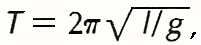Definition: pendulum from Dictionary of Energy

Physics. any body that is free to swing under the influence of gravity; a simple practical example is a small weight suspended from the end of a string.

Summary Article: pendulum
From The Columbia Encyclopedia

a mass, called a bob, suspended from a fixed point so that it can swing in an arc determined by its momentum and the force of gravity. The length of a pendulum is the distance from the point of suspension to the center of gravity of the bob (see center of mass). Chance observation of a swinging church lamp led Galileo to find that a pendulum made every swing in the same time, independent of the size of the arc. He used this discovery in measuring time in his astronomical studies. His experiments showed that the longer the pendulum, the longer is the time of its swing. Christiaan Huygens determined an exact relation between the length of the pendulum and the time of vibration when the arc of swing is small. He arrived at the formulawhere T is the period, or time for one complete swing, l is the length, g is the acceleration of gravity (see gravitation), and π = 3.14 … (see harmonic motion). In 1673, Huygens devised a practicable means of making a pendulum control the speed with which a clock mechanism runs. This impetus to clockmaking resulted not only in the development of many types of clock, such as the “wag at the wall,” the grandfather clock, and the banjo clock, but also in the application of pendulum control to other mechanisms. Metal pendulums are lengthened by heat; to counteract the effect of temperature changes, compensation pendulums have been devised, many of them operating by the opposite expansion of different metals in compound rods. Forces acting on the bob, such as air resistance, affect its swing. Since gravity varies from place to place, the pendulum has been used to determine the shape and mass of the earth by measuring the intensity of gravity. In the seismograph (see seismology), a pendulum registers the direction of an earthquake. In 1851, J. B. L. Foucault demonstrated the rotation of the earth by suspending in the Panthéon in Paris a 200-ft (61-m) pendulum that traced its path in sand on the floor. The pendulum continued to vibrate in a single plane as the earth rotated underneath it, thus leaving a series of traces in the sand in all directions. A pendulum made to swing in a circle, describing a cone, is called a conical pendulum. In the torsion pendulum the bob vibrates by twisting and untwisting, as in the balance wheel of a watch.The Columbia Encyclopedia, © Columbia University Press 2018

Related Articles

Full text Article pendulum
Chambers Dictionary of the Unexplained

A weight suspended from a thread or string so that it can swing freely, used for divination or dowsing. One of the tools most widely used...

See more from Credo